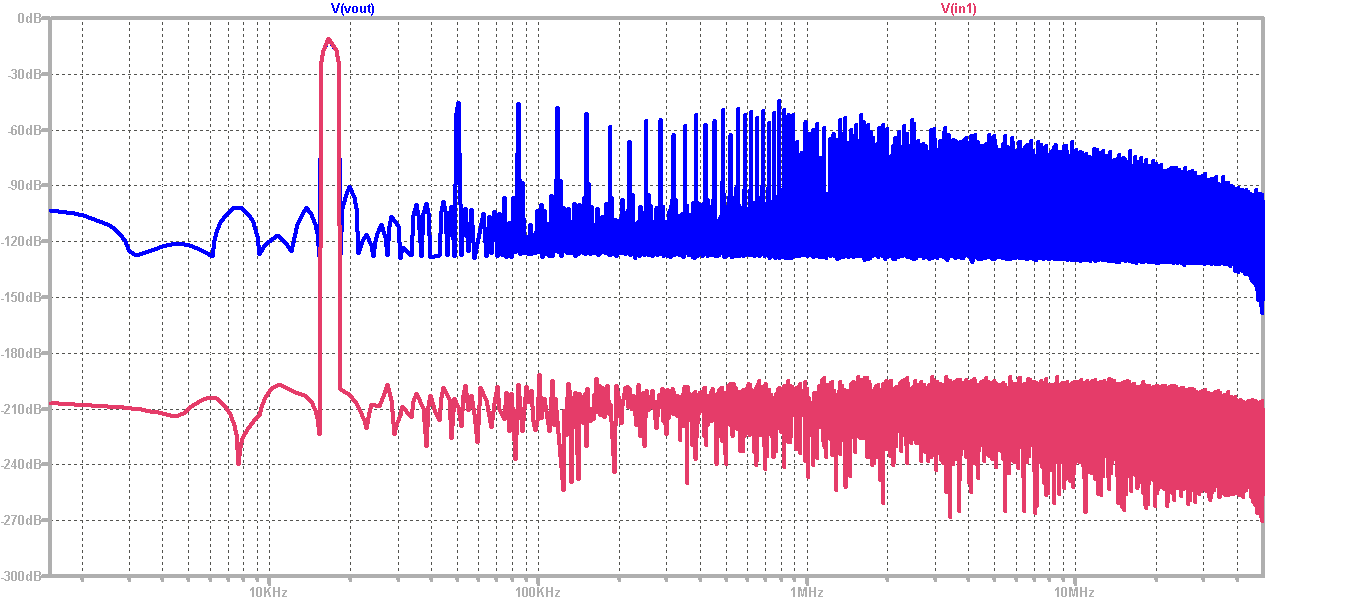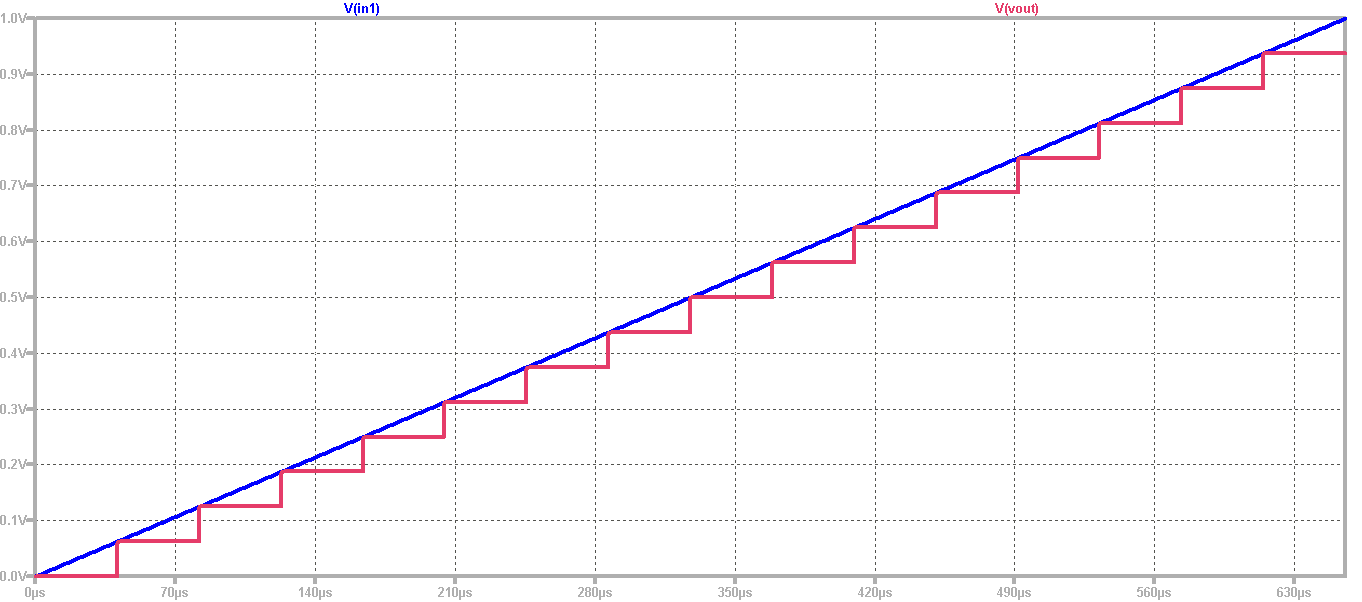# Overview

• Analysis of 4 bit ADC & DAC

• ADC DAC Schematic
• Simulation and Analysis of a 4 bit ADC & DAC for a Sine signal
• Simulation and Analysis of a 4 bit ADC & DAC for a Ramp signal
• R2R DAC

• Schematic of R2R DAC
• Simulation and Analysis of R2R DAC for a Sine Signal
• Simulation and Analysis of R2R DAC for a Ramp Signal
• Summary and Learning Outcomes

# ADC DAC Schematic

The schematic of a 4 bit ADC and DAC has been shown below.

Ideal components have been used in this circuit in order to get an output almsot similar to the input.

# Simulation of a 4 bit ADC & DAC for a Sine signal

A Sine wave has been simulated for a 4 bit ADC and DAC with ideal components.

The output analogue wave can be seen in the red in the simulation given below.

A Sine wave with 11 periods can be seen in the figure given below.# Fast Fourier Transform for a Sine signal

A Fast Fourier transform for a sine signal can be seen in the given figure.

The signal to noise levels has been observed and the input has better Signal to noise level because it is just a 4 bit converter.

We need external data processors because LTSPICE doesn't provide with all the values.# Schematic and Simulation of a 4 bit ADC & DAC for a Ramp signal

 A Ramp response for a 4 Bit ADC and DAC has been observed in LTSPICE. A source has been added for this purpose. V1 in1 0 PULSE(0 1 0 655.36u 655.36u 0 1310.72u)# INL and DNL Analysis for a Ramp Signal

For Filteration, we have used an online Javascript tool.

The tool can be accessed using the Read Raw File given at the webpage of Lab 3 of Interface Electronics.

Then, raw.file was used for further analysis.# Histogram Test of ADC for a Ramp Signal

I got the results given below by again using the Javascript tool.

For this test, I increased the number of steps, in order to see how many times each digital code word appears on the output of ADC.

INL and DNL are zero because of the ideal case.# INL and DNL Analysis for a Sine Signal

I got the INL and DNL by again using the Javascript tool.

The graph on the left side shows the INL and DNL for the sine signal.

The graph on the right side shows the extracted values.# FFT Data Analysis for a Sine Signal

For a better analysis, I did the Fast Fourier Transform using the FFT webpage provided.

I also also observed the Signal to Noise Ratio (SNR).

The graph on the upper right corner shows the FFT for the Sine Signal.

The grpah on the lower right corner shows the histogram test.# Schematic of R2R DAC

The schematic for the R2R DAC can be seen below.

The resistance values has been changed in this circuit for R6 and R9 for further analysis.

# Simulation and Analysis of R2R DAC for a Sine Signal

You can see the FFT for R2R DAC for a Sine Signal on the upper right side.

You can see the variations in INL and DNL and it is not ideal because we changed the resistance values.

The change of resistance values and corresponding INL/DNL values depicts that any minor change can be observed by having a look at INL and DNL graph.# Simulation and Analysis of R2R DAC for a Ramp Signal

The INL and DNL graph can be seen at the right side of the figure given below.

The graph on the left side shows the effect of each individual change of resistance, the variation in the middle corresponds to change of R9 value.

The variation depends on the order of the bit.# Summary and Learning Outcomes

• In this laboratory, We went into more details of Analog and Digital conversion and vice versa.
• We did analysis by observing the DNL, INL, FFT for the Ramp and the Sine signal.
• By using the Histogram test, we verified the integral (INL) and differential (DNL) non-linearity specifications.
• Then, we implemented the R2R circuit and observed its response as well.
• By observing the INL and DNL graph, we were able to identify any change in the resistance values.
• This laboratory helped to be more efficient in LTSPICE as well.
• With the help of page source option of Firefox, I learnt a number of new features of HTML which I implemented in this web report as well.
• It was very time consuming, but after all the learning I can say it was worth it.

# References

 Making of a Webreport , Vollrath

 Laboratory 03: ADC DAC analysis instructions , Vollrath